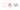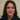# Javascript ES6 modern rest parameters are explained with examples

We have covered spread syntax in an article before and here we will explain Javascript ES6 modern rest parameter in this article.

## The rest parameters syntax

According to MDN A function’s last parameter can be prefixed with `...`  which makes the last parameter a “rest” parameter .

Then we have all remaining arguments to be placed within “standard” javascript array. Only the last parameter can be a “rest parameter”:

``````function f(a, b, ...theArgs) {
// ...
}<br/><br/>// Using <a href="https://www.nikpro.com.au/all-you-need-to-know-about-arrow-functions-in-javascript/">arrow functions<br/><br/></a>const f = (a,b,  ...theArgs) => {<br/> // ...
}``````

Technically we need to know that the `arguments` object is not a real array, while rest parameters are `Array`instances, meaning methods like `sort``map``forEach` or `pop` can be applied on it directly;### Destructuring rest parameters

Basically we can destructure an array using rest parameters in different ways.Take a look at some examples. I use arrow function syntax in these examples:

``````const myFun = (a, b, …manyMoreArgs) => {
console.log("a", a);
console.log("b", b);
console.log("manyMoreArgs", manyMoreArgs);
}

myFun("one", "two", "three", "four", "five", "six");

// a, one
// b, two
// manyMoreArgs, [three, four, five, six]``````

As an example we have …manyMoreArgs as rest parameters. Therefor whatever argument to be assigned after the first two arguments will be part of the rest parameters:

``````// using the same function definition from example above

myFun("one", "two", "three");

// a, one
// b, two
// manyMoreArgs, [three]<br/><br/>// Another call<br/><br/>myFun("one", "two");

// a, one
// b, two
// manyMoreArgs, []``````

Although in the last function call the last parameter wasn’t specified but we still have an array which is empty.

### Using array methods on rest parameters

AS I mentioned above we could use array methods on rest parameter args because it is an array. Take a look at these example:

``````const sortRestArgs = (...theArgs) => {
return theArgs.sort();
}

console.log(sortRestArgs(5, 3, 7, 1)); // 1, 3, 5, 7``````

But we cannot do the same with arguments as they are not an array:

``````// This is wrong and will not work. <br/>const sortArguments = (arguments) => {
return arguments.sort();
}``````

Best approach is to convert it to an array and then use array methods:

``````const sortArguments = (arguments) => {
const args = Array.from(arguments);
return args.sort();
}

console.log(sortArguments(5, 3, 7, 1)); // 1, 3, 5, 7``````

We demonstrated rest parameter syntax which allows us to represent an indefinite number of arguments as an array and we can get advantage of array methods using them.